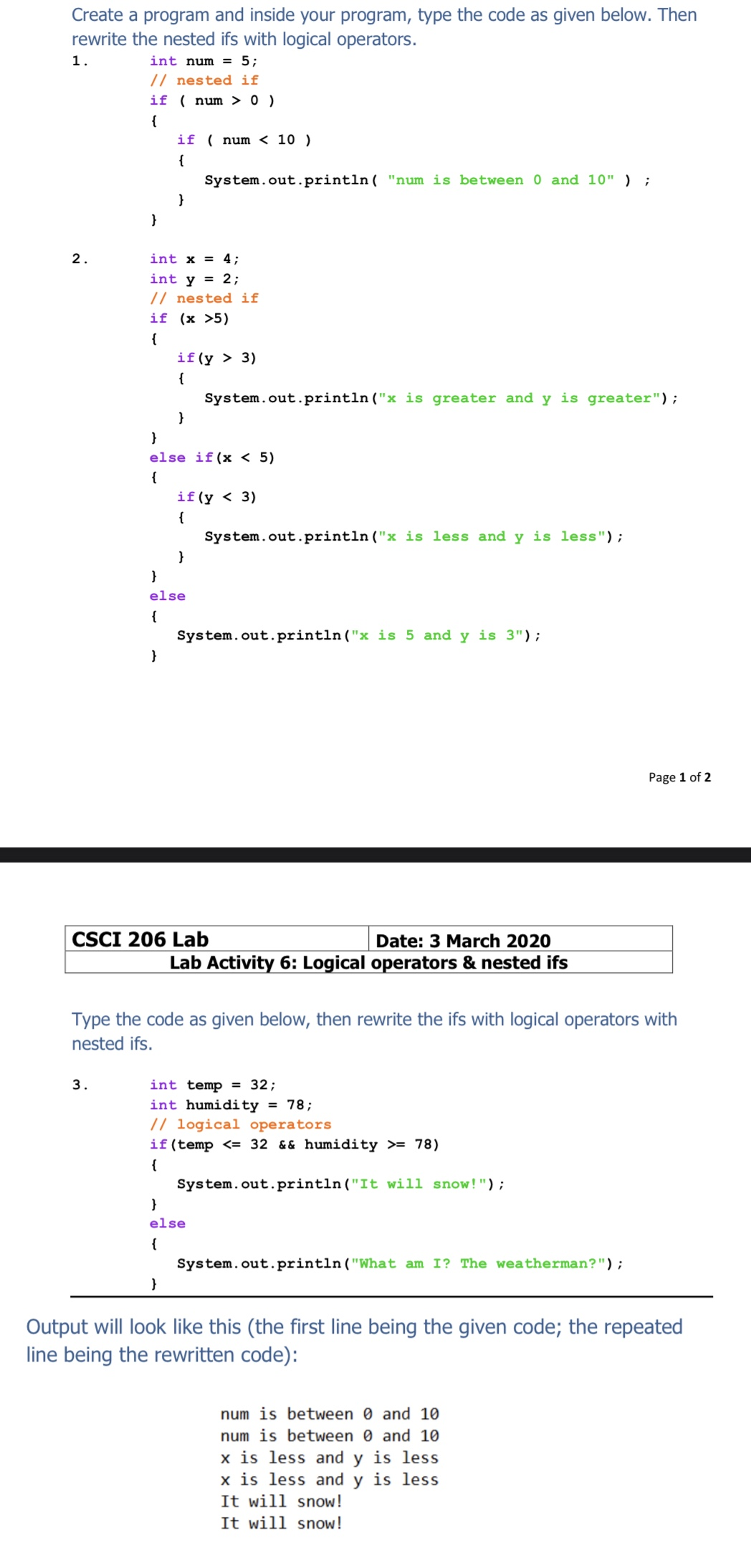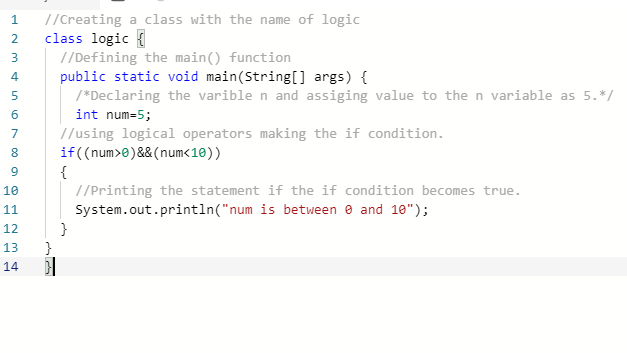# Create a program and inside your program, type the code as given below. Thenrewrite the nested ifs with logical operators.1.int num = 5;// nested ifif ( num > 0 ){if ( num < 10 ){System.out.println( "num is between 0 and 10" ) ;}2.int x = 4;int y = 2;// nested ifif (x >5){if (y > 3){System.out.println ("x is greater and y is greater");else if(x < 5){if (y < 3){System.out.println ("x is less and y is less");elseSystem.out.println ("x is 5 and y is 3");Page 1 of 2CSCI 206 LabDate: 3 March 2020Lab Activity 6: Logical operators & nested ifsType the code as given below, then rewrite the ifs with logical operators withnested ifs.3.int temp = 32;int humidity = 78;// logical operatorsif(temp = 78){System.out.println("It will snow!");else{System.out.println ("What am I? The weatherman?");Output will look like this (the first line being the given code; the repeatedline being the rewritten code):num is between 0 and 10num is between 0 and 10x is less and y is lessx is less and y is lessIt will snow!It will snow!

Question
18 viewshelp_outlineImage TranscriptioncloseCreate a program and inside your program, type the code as given below. Then rewrite the nested ifs with logical operators. 1. int num = 5; // nested if if ( num > 0 ) { if ( num < 10 ) { System.out.println( "num is between 0 and 10" ) ; } 2. int x = 4; int y = 2; // nested if if (x >5) { if (y > 3) { System.out.println ("x is greater and y is greater"); else if(x < 5) { if (y < 3) { System.out.println ("x is less and y is less"); else System.out.println ("x is 5 and y is 3"); Page 1 of 2 CSCI 206 Lab Date: 3 March 2020 Lab Activity 6: Logical operators & nested ifs Type the code as given below, then rewrite the ifs with logical operators with nested ifs. 3. int temp = 32; int humidity = 78; // logical operators if(temp <= 32 && humidity >= 78) { System.out.println("It will snow!"); else { System.out.println ("What am I? The weatherman?"); Output will look like this (the first line being the given code; the repeated line being the rewritten code): num is between 0 and 10 num is between 0 and 10 x is less and y is less x is less and y is less It will snow! It will snow! fullscreen
check_circle

Step 1

Hey, since there are multiple questions posted, we will answer first question. If you want any specific question to be answered then please submit that question only or specify the question number in your message.

Step 2

PROGRAM:

//Creating a class with the name of logic

class logic

{

//Defining the main() function

public static void main(String[] args)

{

/*Declaring the variable n and assigning value to the n variable as 5.*/

int num=5;

//using logical operators making the if condition.

if((num>0)&&(num<10))

{

//Printing the statement if the condition becomes true.

System.out.println("num is between 0 and 10");

}

}

}

Step 3

SREENSHOT OF THE PROGRAM:...

### Want to see the full answer?

See Solution

#### Want to see this answer and more?

Solutions are written by subject experts who are available 24/7. Questions are typically answered within 1 hour.*

See Solution
*Response times may vary by subject and question.
Tagged in